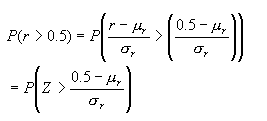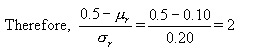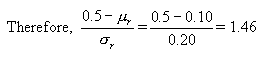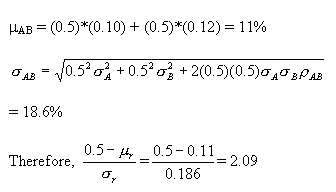# Probability of One Portfolio Outperforming Another Portfolio

Let us consider two assets A and B with the following details:

MeanStandard DeviationCorrelation
A$$\mu_{A}=10\%$$$$\sigma_{A}=20\%$$$$\rho_{AB}=30\%$$
B$$\mu_{B}=12\%$$$$\sigma_{B}=26\%$$

We have a total of $10 million to invest. Our objective is to reach a target return of$5 million. Let us look at the following three options and find out the probability of reaching our target in each case:

1. Entire $10 million in Asset A 2. Entire$10 million in Asset B
3. $5 million in A and$5 million in B

Assuming r as the return from each portfolio, our objective can be expressed as follows:

P (10million*r > 5million)

Or

P (r > 0.5)

We know that if the returns of an asset are normally distributed, it can be expressed as a function of standard normal distribution. We can associate the return distribution to a standard normal distribution, which has a zero mean and a standard deviation of one.

Therefore, our returns can be expressed as follows:

$$r = \mu{r} + Z\sigma{r}$$

or $$Z = (r - \mu{r})/ \sigma{r}$$

Our probability can be expressed as follows:Let us now evaluate each of the three portfolios.

1. Entire $10 million in Asset A $$\mu_{A}=10\%$$ $$\sigma_{A}=20\%$$That is, P(Z > 2) = 2.28% 2. Entire$10 million in Asset B

$$\mu_{B}=12\%$$

$$\sigma_{B}=26\%$$That is, P(Z > 1.46) = 7.19%

3. $5 million in A and$5 million in B

First we need to calculate the expected return and standard deviation of the portfolio.That is, P(Z > 2.09) = 1.8%

### Conclusion

You can observe that the portfolio with the highest risk has the greatest chance of reaching the target, while the diversified portfolio has the least chance of reaching the target.